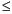Functions and CALL Routines

# PROBHYPR Function

Returns the probability from a hypergeometric distribution.
 Category: Probability See: CDF Function

## Syntax

 PROBHYPR(N,K,n,x<,r>)

### Arguments

N

is an integer population size parameter, with N1.

 Range:
K

is an integer number of items in the category of interest parameter.

 Range: 0KN
n

is an integer sample size parameter.

 Range: 0nN
x

is an integer random variable.

 Range: max(0, K + n-N)xmin(K,n)
r

is an optional numeric odds ratio parameter.

 Range: r0

The PROBHYPR function returns the probability that an observation from an extended hypergeometric distribution, with population size N, number of items K, sample size n, and odds ratio r, is less than or equal to x. If the optional parameter r is not specified or is set to 1, the value returned is from the usual hypergeometric distribution.

SAS Statements Results
`x=probhypr(200,50,10,2);`
`0.5236734081`

 Functions:Previous Page | Next Page | Top of Page